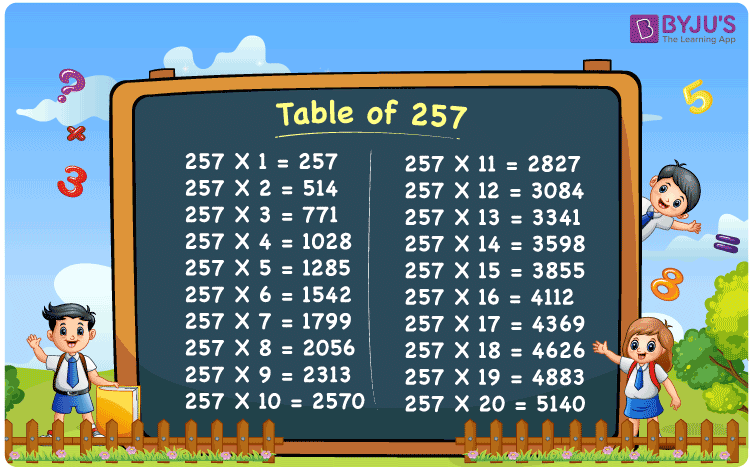Checkout JEE MAINS 2022 Question Paper Analysis : Checkout JEE MAINS 2022 Question Paper Analysis :

# Table of 257

The Table of 257 is the multiplication table that includes the values produced on multiplying 257 by whole numbers. 257 times table represents the multiples when the original number is multiplied by consecutive natural numbers. This table can also be expressed in repeated addition form, where 257 is added to itself to get its multiples. For example, 257 multiplied by 3 is: 257 + 257 + 257 = 771 = 257 x 3. Learn more about Multiplication tables at BYJU’S and download the PDF of Table of 257 from the below-given link.

## Table of 257 Chart## What is the 257 Times Table?

Learn how to write the 257 times table, with the help of the repeated addition table given below.

 257×1 = 257 257 257×2 = 514 257 + 257 = 514 257×3 = 771 257 + 257 + 257 = 771 257×4 = 1028 257 + 257 + 257 + 257 = 1028 257×5 = 1285 257 + 257 + 257 + 257 + 257 = 1285 257×6 = 1542 257 + 257 + 257 + 257 + 257 + 257 = 1542 257×7 = 1799 257 + 257 + 257 + 257 + 257 + 257 + 257 = 1799 257×8 = 2056 257 + 257 + 257 + 257 + 257 + 257 + 257 + 257 = 2056 257×9 = 2313 257 + 257 + 257 + 257 + 257 + 257 + 257 + 257 + 257 = 2313 257×10 = 2570 257 + 257 + 257 + 257 + 257 + 257 + 257 + 257 + 257 + 257 = 2570

## Multiplication Table of 257

Here is the table of 257 up to 20 times below.

 257 × 1 = 257 257 × 2 = 514 257 × 3 = 771 257 × 4 = 1028 257 × 5 = 1285 257 × 6 = 1542 257 × 7 = 1799 257 × 8 = 2056 257 × 9 = 2313 257 × 10 = 2570 257 × 11 = 2827 257 × 12 = 3084 257 × 13 = 3341 257 × 14 = 3598 257 × 15 = 3855 257 × 16 = 4112 257 × 17 = 4369 257 × 18 = 4626 257 × 19 = 4883 257 × 20 = 5140

## Solved Examples on Table of 257

Q.1: Find the value of 2000 – 257 x 5.

Solution: Given,

2000 – 257 x 5

From the table of 257, we have;

257 x 5 = 1285

Hence,

= 2000 – 1285

= 715

Q.2: John bought a plant for Rs. 257. He wants to buy the same plant to put it at 4 different corners of his balcony. What is the total purchase he made?

Solution: Given,

Price of a plant = Rs. 257

Price of 4 such plants = Rs. 257 x 4

= Rs. 1028

## Frequently Asked Questions – FAQs

### What is table of 257?

Table of 257 is the multiplication table that represents the product of the original number with a set of positive integers.

### How to write 257 times table?

257 times table can be written as:

257 x 1 = 257

257 x 2 = 514

257 x 3 = 771

257 x 4 = 1028

257 x 5 = 1285

And so on.

### What should be multiplied by 257 to get 1799?

From the table of 257, when we multiply 257 by 7 the resulting value is 1799.

### What is the value of 257 x 12?

From the table of 257, we have 257 x 12 = 3084